# Appex 07 – Linear Regression in R

STA 363 - Spring 2023

## Set up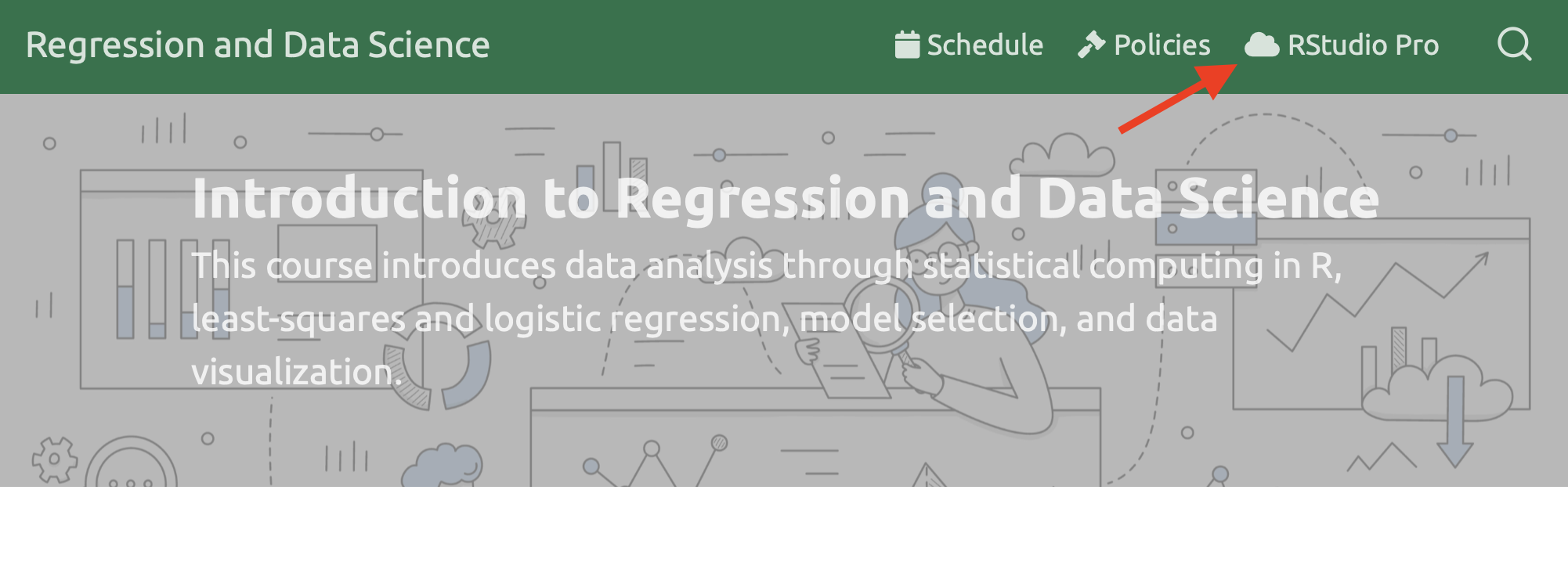#### Step 1: Create a New Project

Click File > New Project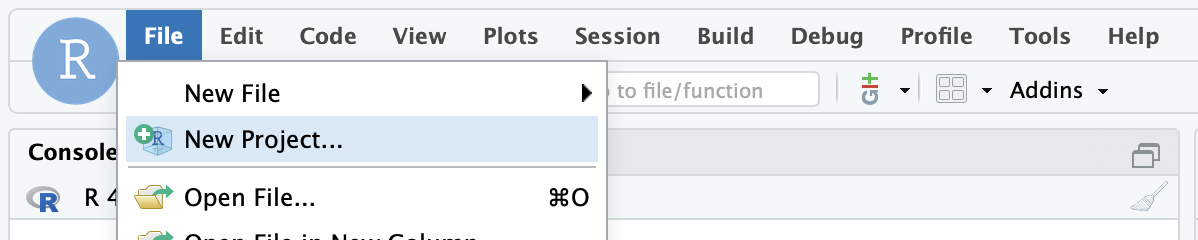#### Step 2: Click “Version Control”

Click the third option.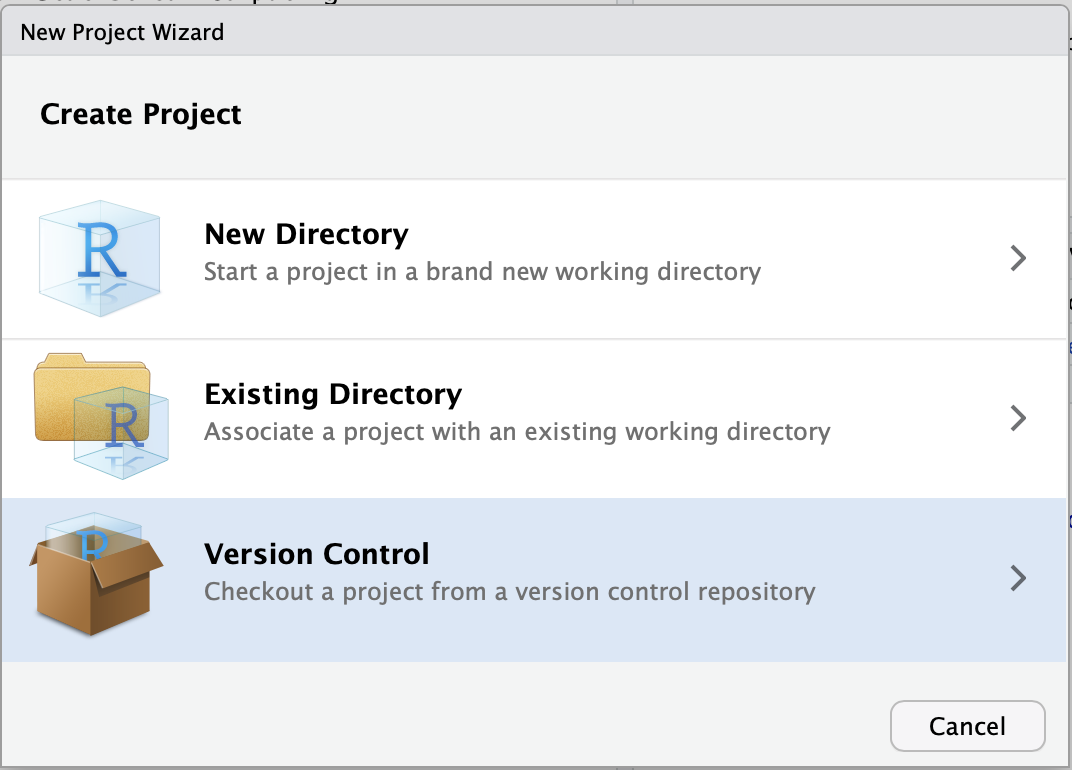#### Step 3: Click Git

Click the first option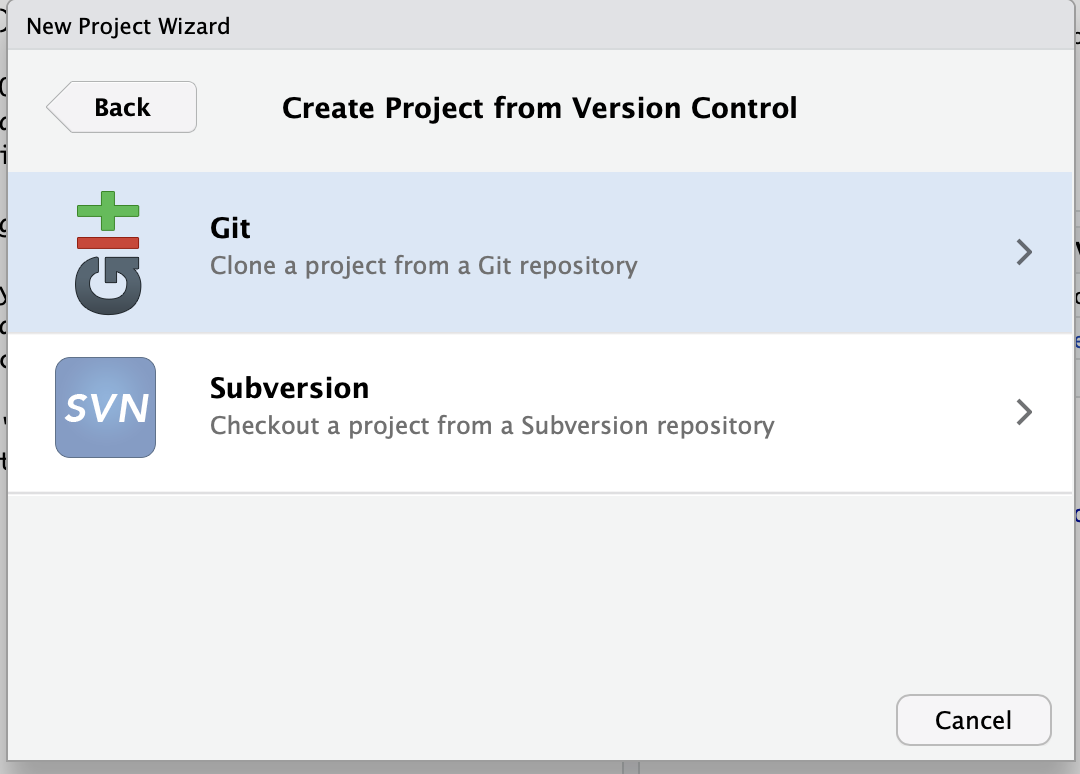#### Step 4: Copy my starter files

Paste this link in the top box (Repository url):

https://github.com/sta-363-s23/08-appex.git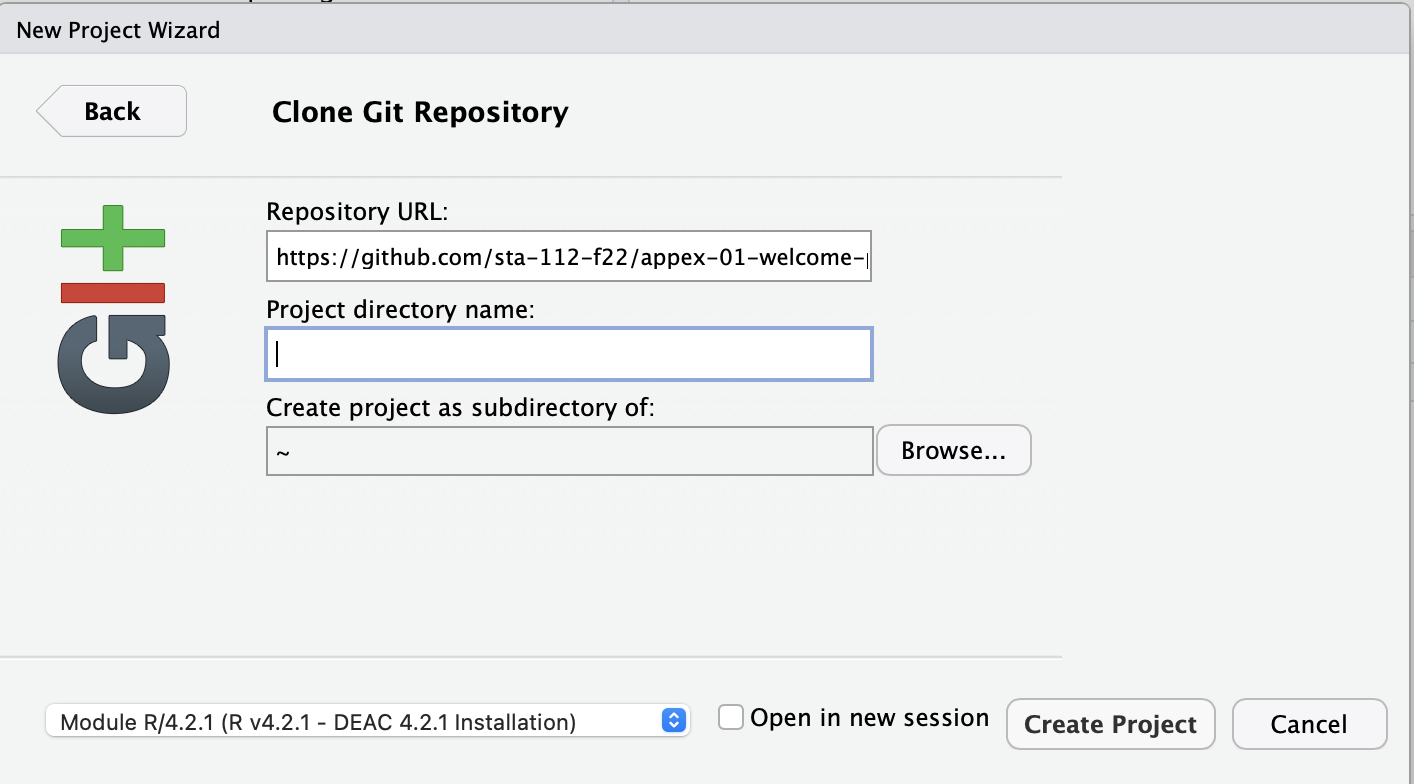## Part 1

1. Fit a linear model using the mtcars data frame predicting miles per gallon (mpg) from weight and horsepower (wt and hp), using polynomials with 4 degrees of freedom for both.
2. Pull out the coefficients and confidence intervals using the tidy() function demonstrated. How do you interpret these?

## Part 2

1. Using the linear model you fit previously (mpg from wt and hp, using polynomials with 4 degrees of freedom for both with the mtcars data) - calculate the p-value for the coefficient for weight
2. Interpret this value. What is the null hypothesis? What is the alternative hypothesis? Do you reject the null?

## Part 3

1. Using the model previously fit (mpg from wt and hp, using polynomials with 4 degrees of freedom for both with the mtcars data), estimate the training $$R^2$$ using the rsq function.
2. Interpret this values.

## Part 4

1. Create a cross validation object to do 5 fold cross validation using the mtcars data
2. Refit the model on this object (using fit_resamples)
3. Use collect_metrics to estimate the test $$R^2$$ - how does this compare to the training $$R^2$$ calculated in the previous exercise?